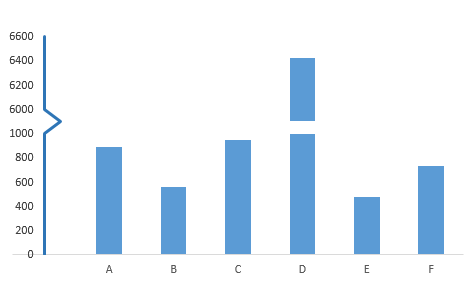# Axis break for graph with polar coordinate

Hi, I was trying to make a histogram in polar coordinate system. However, one category has a large amount of datapoint (>1000) whereas the other categories have significantly fewer points (<280). The graph turns out to be very hard to read. See below:

I wonder if I can add an axis break on the graph to make it nicer, like this:I tried to manipulate the range of the axis but then it failed. And this is my code right now:

``````fig <- plot_ly(type = 'scatterpolar', r = df\$Freq, fill = 'toself')  %>%
add_trace(type = 'scatterpolar', r = df\$Freq, fill = 'toself') %>%
add_trace(type = 'scatterpolar', r = df\$Freq, fill =  'toself') %>%
add_trace(type = 'scatterpolar', r = df\$Freq, fill =  'toself') %>%
layout(polar = list(radialaxis = list(visible = T,range = c(0,1300))))
``````

Could anyone help me?
Thank you for helping!!!